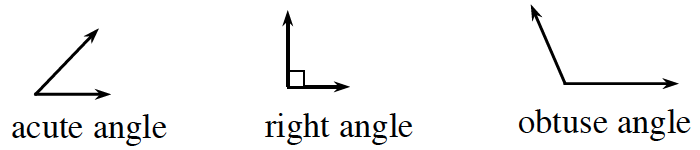### Home > CCAA > Chapter 8 Unit 7 > Lesson CC2: 8.3.3 > Problem8-86

8-86.

Use a straightedge to draw a triangle on your paper.  Homework Help ✎

1. Estimate the measure of each angle in your triangle and label it.

Refer to the Math Notes box excerpt below.
Can you estimate your angle measures by comparing them to the examples below?
Be sure your work is neat!

 Angles are named by whether they are less than, greater than, or equal to a right angle. An acute angle measures less than $90°$. An obtuse angle measures more than $90°$ and less than $180°$.2. Add your three estimated angle measures. What do you notice? Do you need to change any of your estimates?

The three angles of a triangle should add up to $180°$. Do your angle estimates follow this rule?